Problem Solving Tool KFC What is Problem Solving

• Slides: 18Problem Solving Tool: KFC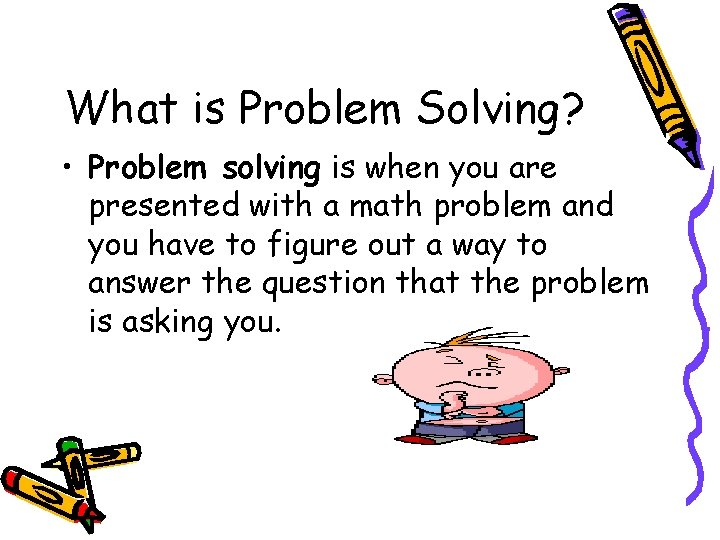What is Problem Solving? • Problem solving is when you are presented with a math problem and you have to figure out a way to answer the question that the problem is asking you.Problem Solving can be tough but not if you use… • K • F • C • SNo, not fried chicken! • K- stands for “What information do I know? ” • F- stands for “What am I trying to find out? ” • C- stands for “Come up with a plan!” • S- stands for “Solve!”The PLAN you come up with must have two parts! 1. What operation will I need to use? Addition, subtraction, division, multiplication, ordering, comparing, and/or estimating …? 2. What problem solving strategy will I choose to use?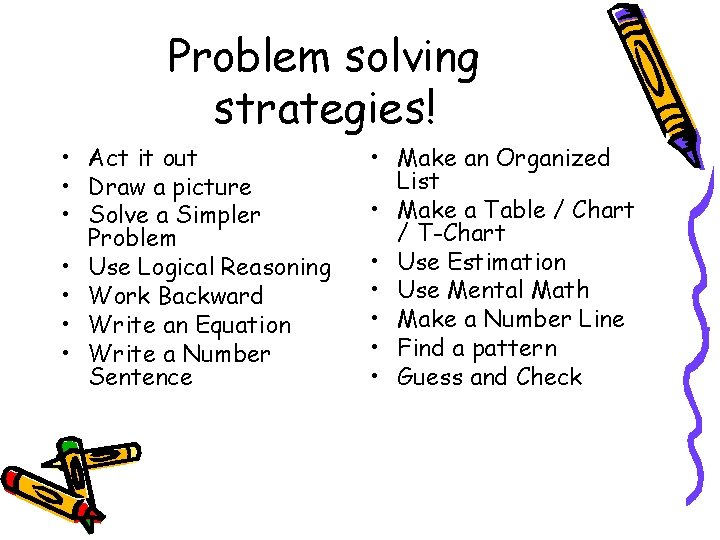Problem solving strategies! • Act it out • Draw a picture • Solve a Simpler Problem • Use Logical Reasoning • Work Backward • Write an Equation • Write a Number Sentence • Make an Organized List • Make a Table / Chart / T-Chart • Use Estimation • Use Mental Math • Make a Number Line • Find a pattern • Guess and CheckLet’s try it! Here’s the problem: • Paul received four postcards from each of his father’s 11 trips. How many postcards did Paul receive from the 11 trips?First is “K” or “What do you know and need to solve the problem? ” • Paul received four postcards from each of his father’s 11 trips. How many postcards did Paul receive from the 11 trips?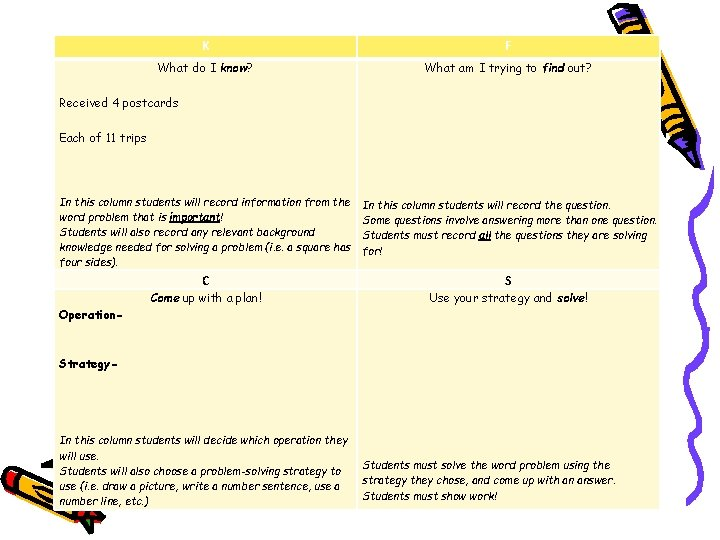K F What do I know? What am I trying to find out? Received 4 postcards Each of 11 trips In this column students will record information from the word problem that is important! Students will also record any relevant background knowledge needed for solving a problem (i. e. a square has four sides). In this column students will record the question. Some questions involve answering more than one question. Students must record all the questions they are solving for! C S Come up with a plan! Use your strategy and solve! Operation- Strategy- In this column students will decide which operation they will use. Students will also choose a problem-solving strategy to use (i. e. draw a picture, write a number sentence, use a number line, etc. ) Students must solve the word problem using the strategy they chose, and come up with an answer. Students must show work!Next is “F” or “What am I trying to find out? ” • Paul received four postcards from each of his father’s 11 trips. How many postcards did Paul receive from the 11 trips?K F What do I know? What am I trying to find out? Received 4 postcards How many postcards did Paul receive from the 11 trips? Each of 11 trips In this column students will record information from the word problem that is important! Students will also record any relevant background knowledge needed for solving a problem (i. e. a square has four sides). In this column students will record the question. Some questions involve answering more than one question. Students must record all the questions they are solving for! C S Come up with a plan! Use your strategy and solve! Operation- Strategy- In this column students will decide which operation they will use. Students will also choose a problem-solving strategy to use (i. e. draw a picture, write a number sentence, use a number line, etc. ) Students must solve the word problem using the strategy they chose, and come up with an answer. Students must show work!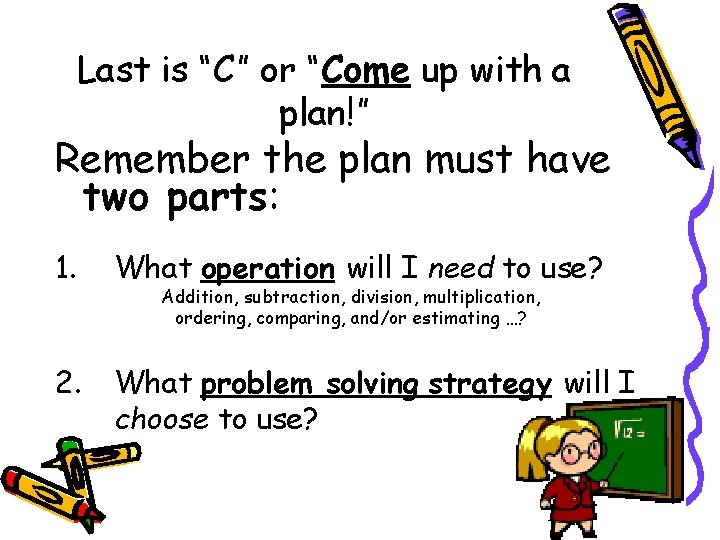Last is “C” or “Come up with a plan!” Remember the plan must have two parts: 1. What operation will I need to use? 2. What problem solving strategy will I choose to use? Addition, subtraction, division, multiplication, ordering, comparing, and/or estimating …?How do we choose an operation? Look for KEY words! Addition add in all total sum plus how much perimeter increased by more than both together all together Subtraction subtract difference how much more decreased by minus less than fewer than have left exceed take away are not remain change left Multiplication multiplied by times each product total area Division divided by share equally part each distribute evenly average out of per quotient split separated cut upK F What do I know? What am I trying to find out? Received 4 postcards How many postcards did Paul receive from the 11 trips? Each of 11 trips In this column students will record information from the word problem that is important! Students will also record any relevant background knowledge needed for solving a problem (i. e. a square has four sides). In this column students will record the question. Some questions involve answering more than one question. Students must record all the questions they are solving for! C S Come up with a plan! Use your strategy and solve! Operation- Multiplication Strategy- Draw a Picture In this column students will decide which operation they will use. Students will also choose a problem-solving strategy to use (i. e. draw a picture, write a number sentence, use a number line, etc. ) Students must solve the word problem using the strategy they chose, and come up with an answer. Students must show work!Now it’s time to solve the word problem!K F What do I know? What am I trying to find out? Received 4 postcards How many postcards did Paul receive from the 11 trips? Each of 11 trips In this column students will record information from the word problem that is important! Students will also record any relevant background knowledge needed for solving a problem (i. e. a square has four sides). In this column students will record the question. Some questions involve answering more than one question. Students must record all the questions they are solving for! C S Come up with a plan! Use your strategy and solve! Operation- Multiplication Strategy- Draw a Picture In this column students will decide which operation they will use. Students will also choose a problem-solving strategy to use (i. e. draw a picture, write a number sentence, use a number line, etc. ) OOOO OOOO OOO Four groups of 11 is 44. 4 x 11 = 44 Paul received 44 postcards.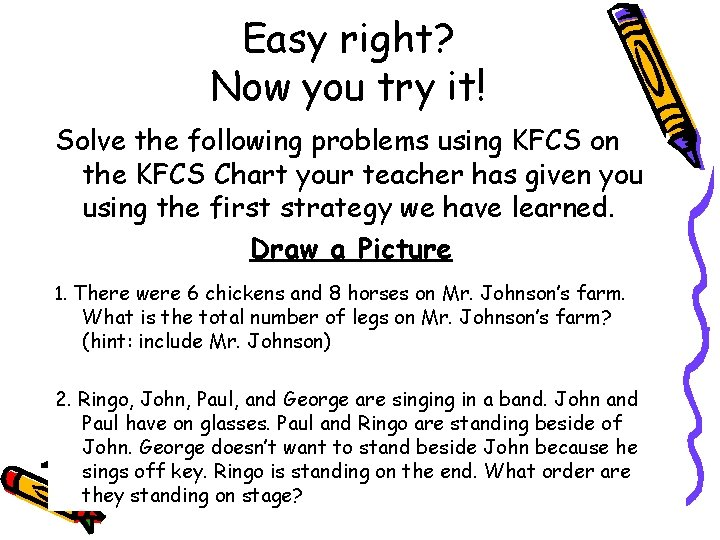Easy right? Now you try it! Solve the following problems using KFCS on the KFCS Chart your teacher has given you using the first strategy we have learned. Draw a Picture 1. There were 6 chickens and 8 horses on Mr. Johnson’s farm. What is the total number of legs on Mr. Johnson’s farm? (hint: include Mr. Johnson) 2. Ringo, John, Paul, and George are singing in a band. John and Paul have on glasses. Paul and Ringo are standing beside of John. George doesn’t want to stand beside John because he sings off key. Ringo is standing on the end. What order are they standing on stage?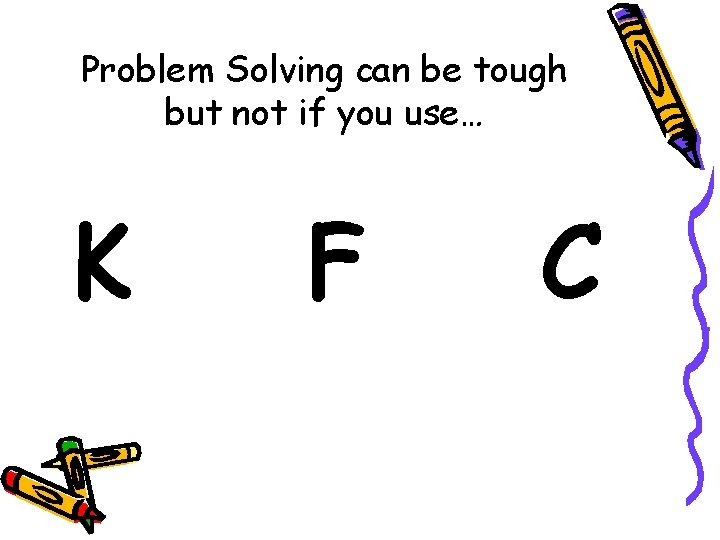Problem Solving can be tough but not if you use… K F C